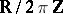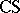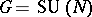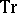Chern-Simons functional

(diff) ← Older revision | Latest revision (diff) | Newer revision → (diff)

A Lagrangian in the theory of gauge fields on an oriented manifoldof dimension. More precisely, it is a-valued functionon the space of connections ( "gauge fields" ) on a principal-bundle (cf. also Principal-object) with base spacefor a compact connected Lie group. Forsimply connected, e.g., the bundle can be taken to be the product bundle and the Chern–Simons functional is given by the formulawhere the connection is given by the matrix-valued-formandis the usual trace of matrices (cf. also Trace of a square matrix).is invariant under gauge transformations, i.e. automorphisms of the-bundle, and hence it defines a Lagrangian on the space of orbits for the action of the group of these. The critical points ofare given by the flat connections, i.e. those for which the curvaturevanishes (cf. also Connection).

Applications of the Chern–Simons functional.

1) Using the Chern–Simons functional as a Morse function, A. Flöer [a6] defined invariants for homology-spheres related to the Casson invariant (see [a7]).

2) E. Witten [a8] used the Chern–Simons functional to set up a topological quantum field theory (cf. also Quantum field theory), which gives rise to invariants for knots and links in-manifolds including the Jones polynomial for knots in the-sphere. See also [a1] and [a2].

The Chern–Simons functional is a special case of the Chern–Simons invariant and characteristic classes. General references are [a3], [a4], [a5].